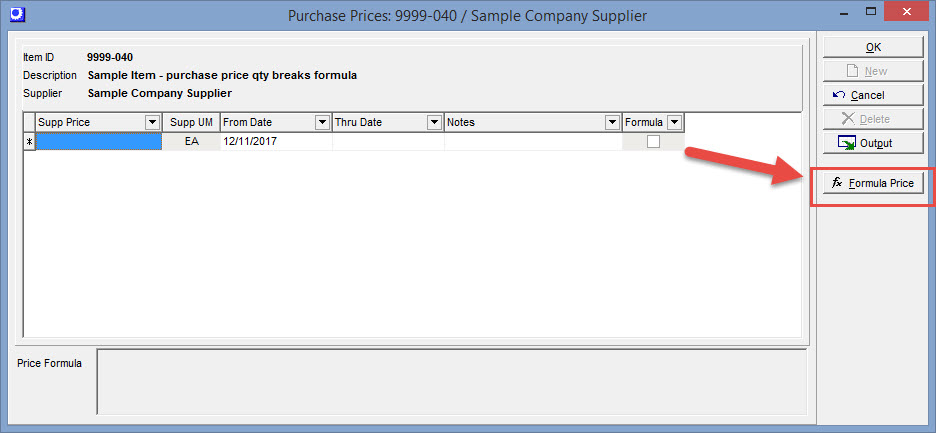## Quantity Price Breaks for Purchase Prices

Formulas can be used to establish pricing rules for purchase prices in the Item Sources - Formula Pricing

Price Formula: Pricing Formulas use the following variables:

qty = Quantity being ordered

result = supplier price in supplier unit of measure and supplier currency

Example: You would like to set up rules to establish price breaks for purchasing from a particular supplier. If you purchase a quantity of less than 10 the purchase price is 100, for quantities 10 to 99 the price is 75, and quantities 100 or greater the purchase price is 50.

Formula:

If qty <10 then result:=100

If qty >= 10 then result:=75

If qty >=100 then result:=50

## Item - Source screen - Create Supplier Item Price Record with Formula